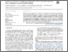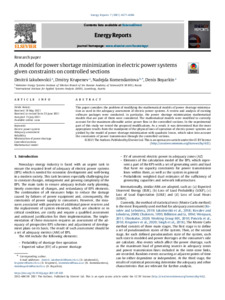A model for power shortage minimization in electric power systems given constraints on controlled sections

Iakubovskii, D., Krupenev, D., , & Boyarkin, D. (2021). A model for power shortage minimization in electric power systems given constraints on controlled sections. Energy Reports 7 4577-4586. 10.1016/j.egyr.2021.07.022.Preview
Text
1-s2.0-S235248472100487X-main.pdf - Published Version

Abstract

This paper considers the problem of modifying the mathematical models of power shortage minimization as used in the adequacy assessment of electric power systems. A review and analysis of existing software packages were conducted, in particular, the power shortage minimization mathematical models that are part of them were considered. The mathematical models were modified to correctly account for the maximum allowable active power flow in the controlled sections. In the experimental part of this study we tested the proposed modifications. As a result, it was determined that the most appropriate results from the standpoint of the physical laws of operation of electric power systems are yielded by the model of power shortage minimization with quadratic losses, which takes into account the constraints of power transmission through the controlled sections.

Item Type: Article Electric power system; Adequacy assessment; Reliability; Minimization of power shortage; Controlled sections; Mathematical model Advancing Systems Analysis (ASA)Advancing Systems Analysis (ASA) > Cooperation and Transformative Governance (CAT) Luke Kirwan 30 Jul 2021 10:29 27 Aug 2021 17:35 http://pure.iiasa.ac.at/17348View Item